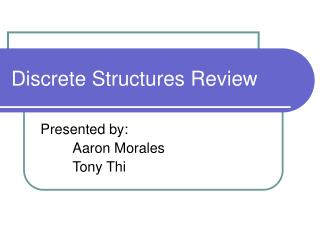Download PresentationDiscrete Structures Review

# Discrete Structures Review - PowerPoint PPT PresentationDownload Presentation## Discrete Structures Review

- - - - - - - - - - - - - - - - - - - - - - - - - - - E N D - - - - - - - - - - - - - - - - - - - - - - - - - - -
##### Presentation Transcript

1. Discrete Structures Review Presented by: Aaron Morales Tony Thi

2. Math Logic • In Math Logic (Propositional Calculus) we use connectives and variables to create statements. • Connectives are:not (negation) ~ ~Xand (conjuction) ^ X ^ Yor (disjunction) V X V YImplies (conditional)  X YIFF (biconditional)  X  Y

3. Truth Tables and Definitions • Two statements are logically EQUIVALENT if they have the same truth table • A Statement is a TAUTOLOGY if its truth table consists entirely of ‘Ts’ • A well formed proposition (WFP) is a valid statement e.g. X ^ Y is WFP, X ^  Y is not

4. Boolean Functions and Logic Gates • Variables are streams of 0s and 1s • True  1 and False  0 • Connective notation changesAND AB OR A + BNOT Ā XOR A (+) B • Logic Gates are icons for boolean operations e.g. NAND, NOR • DeMorgan’s Law: ____ __ ĀĒ = A + E AE = Ā + Ē

5. Elementary Combinatorics • Fundamental Principle of counting • How many 3 digit odd numbers are there that are less than 700? • Unordered samples w/o repetition, is given by the binomial coefficient n over k (n / k) calculated by n! / k!(n-k!).e.g. How many 8-bit binary numbers contain exactly 3 ones? (8/3) = 56 notice 2^8 = 256 • Unordered samples w/repetition, is given by the binomial coefficient n+k-1 over k, (n+k-1 / k)e.g. suppose we have four different donuts, in how many different ways can we select a dozen unordered w/repetition? n=4, k=12, (15 / 12) = (15 / 3) = 455

6. Graph Theory • A graph is a set of points (vertices) together with a set of lines (edges) joining some of these points. • The degree of a vertex is the number of edges connected to it. • A cycle is the path ABC • Graph 2 is a tree, connected and no loops

7. MinCost Spanning Tree • Given a connected graph G, a spanning tree, T, T subset G • A MCST for a graph is a spanning tree for which the sum of the cost is a minimum • By Prim’s Algorithm-Start at random- Choose the smallest cost-Connect an edge that is not in the tree but is the smallest coste.g. 6+2+4+5+5 = 22 • Dijkstra’s Algorithm?

8. Binary Tree • A binary tree is a directed graph (downwards) in which- the in-degree of exactly one vertex (root) is zero- the in-degree of all other vertices is 1- the out-degree of each vertex is either 0, 1, or 2 • What is a sub tree of a binary tree? • What is Pre-Order Search? RoLR • What is Post-Order Search? LRRo

9. Textbook • Introduction to Discrete Mathematicsby McEliece, Ash, and Ash(Random House Publishers)